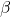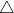# Improved estimation of the parameters of simple linear regression model with autocorrelated errors

The problem of estimating the parameters of a simple linear regression model with
known autocorrelated errors is the main concern of this paper. We consider the
unrestricted estimator, restricted estimator, preliminary test estimator (PTE)
and shrinkage estimator (SE) of the parametersand. The bias and MSE
expressions of the proposed estimators are given and analyzed. From the study
of some graphs and efficiency tables, it is evident that the proposed preliminary
test and shrinkage estimators dominate the unrestricted estimator for some values
of. Sample tables of maximum/minimum efficiencies are provided for variousand level of significance values to assess the performance of the estimators and
choose optimum levels of significance of the PTE. For small size of the test, the
SE performs better than the PT estimator, while for larger sizes, the PT performs
better than the SE.

Fulltext